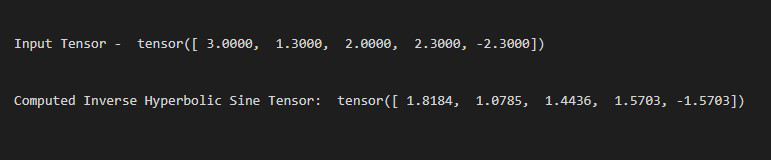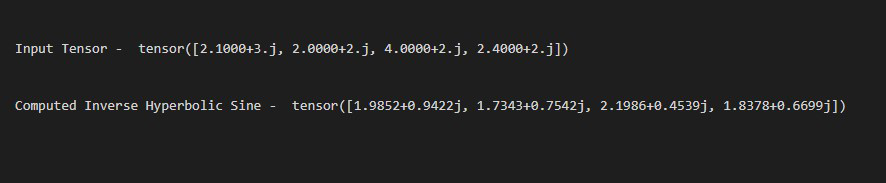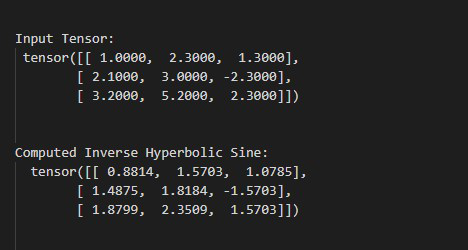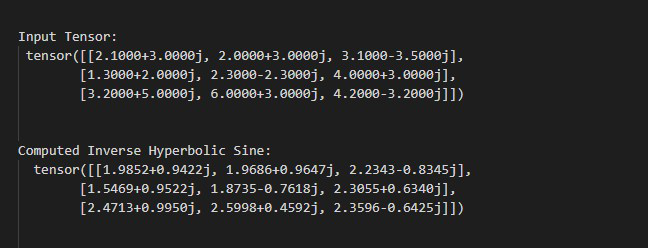# How to compute the inverse hyperbolic sine in PyTorch?

• Last Updated : 27 Jun, 2022

In this article, we are going to discuss how to compute the inverse hyperbolic sine in PyTorch.

## torch.asinh() method:

The torch.asinh() method is used to compute the inverse hyperbolic sine of each element present in a given input tensor. This method accepts both real and complex-valued as input. It supports input tensors of any dimension. This method returns a tensor after computing the inverse hyperbolic sine of each element in a given input tensor. before moving further let’s see the syntax of this method.

Syntax: torch.asinh(input, *, out=None)

Parameters:

• input: This is our input tensor.
• out (optional) – This is our output tensor.

Return: This method returns a tensor after computing the inverse hyperbolic sine of each element in a given input tensor.

Example 1:

In this example, we are computing the inverse hyperbolic sine for the real-valued 1D tensor.

## Python3

 `# Import required library ` `import` `torch ` ` `  `# creating a input tensor ` `tens ``=` `torch.tensor([``3.``, ``1.3``, ``2.``, ``2.3``, ``-``2.3``]) ` ` `  `# print the input tensor ` `print``(``" Input Tensor - "``, tens) ` ` `  `# compute the inverse hyperbolic sine  ` `# of input tensor ` `tens_inv_hsin ``=` `torch.asinh(tens) ` ` `  `# print the above computed tensor ` `print``(``" Computed Inverse Hyperbolic Sine Tensor - "``,  ` `      ``tens_inv_hsin) `

Output:Example 2:

In this example, we are computing the inverse hyperbolic sine for the complex-valued 1D tensor.

## Python3

 `# Import required library ` `import` `torch ` ` `  `# creating a input tensor ` `tens ``=` `torch.tensor([``2.1``+``3j``, ``2.``+``2.j``, ``4.``+``2.j``, ``2.4``+``2.j``]) ` ` `  `# print the input tensor ` `print``(``" Input Tensor - "``, tens) ` ` `  `# compute the inverse hyperbolic sine  ` `# of input tensor ` `tens_inv_hsin ``=` `torch.asinh(tens) ` ` `  `# print the above computed tensor ` `print``(``" Computed Inverse Hyperbolic Sine - "``, ` `      ``tens_inv_hsin) `

Output:Example 3:

In this example, we are computing the inverse hyperbolic sine for the real-valued 2D tensor.

## Python3

 `# Import required library ` `import` `torch ` ` `  `# define a 2D input tensor ` `tens ``=` `torch.tensor([[``1.``, ``2.3``, ``1.3``], ` `                     ``[``2.1``, ``3.``, ``-``2.3``], ` `                     ``[``3.2``, ``5.2``, ``2.3``]]) ` ` `  `# print the input tensor ` `print``(``"\n Input Tensor: \n"``, tens) ` ` `  `# compute the inverse hyperbolic sine of  ` `# input tensor ` `tens_inv_hsin ``=` `torch.asinh(tens) ` ` `  `# print the above computed tensor ` `print``(``"\n Computed Inverse Hyperbolic Sine: \n "``,  ` `      ``tens_inv_hsin) `

Output:Example 4:

In this example, we are computing the inverse hyperbolic sine for the complex-valued 2D tensor.

## Python3

 `# Import required library ` `import` `torch ` ` `  `# define a 2D input tensor ` `tens ``=` `torch.tensor([[``2.1``+``3j``, ``2.``+``3.j``, ``3.1``-``3.5j``], ` `                     ``[``1.3``+``2j``, ``2.3``-``2.3j``, ``4.``+``3.j``], ` `                     ``[``3.2``+``5j``, ``6.``+``3.j``, ``4.2``-``3.2j``]]) ` ` `  `# print the input tensor ` `print``(``"\n Input Tensor: \n"``, tens) ` ` `  `# compute the inverse hyperbolic sine ` `# of input tensor ` `tens_inv_hsin ``=` `torch.asinh(tens) ` ` `  `# print the above computed tensor ` `print``(``"\n Computed Inverse Hyperbolic Sine: \n "``,  ` `      ``tens_inv_hsin)`

Output:My Personal Notes arrow_drop_up
Recommended Articles
Page :